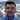# WebStorm 10 and TypeScript support

April 10, 2015

WebStorm 10 now has updated TypeScript support including a built-in TypeScript 1.4 compiler with TypeScript 1.5 support already in the WebStorm 10.0.2 EAP.  To test this out, I opened a simple, sample project where I was playing with JavaScript decimal libraries to understand how certain compiler errors are reported.

I have a TypeScript source code file with intentional compile errors named `bad.ts`

``````/// <reference path="decimal.d.ts" />
var a:Decimal, b:Decimal, c:Decimal;
a = 0.1;
b = 0.2;
c = a + b;``````

It uses the following type definition file which disallows direct assignment of JavaScript numbers or using the `+` operator.  The `Decimal` constructor and `plus()` method are required to be used instead.

``````declare class Decimal {
constructor(value: number);
plus(n: number): Decimal;
plus(n: string): Decimal;
plus(n: Decimal): Decimal;
}``````

`bad.ts` generates these compile time errors:

What is the right way to use this library?  Here is some sample code that avoids the compile time errors.

``````/// <reference path="decimal.d.ts" />
var a:Decimal, b:Decimal, c:Decimal;
a = new Decimal(0.1);
b = new Decimal(0.2);
c = a.plus(0.2);
c = a.plus('0.2');
c = a.plus(b);``````

For more background on why I find this interesting, you can read my earlier blog post: Solution to JavaScript’s 0.1 + 0.2 = 0.30000000000000004 problem?Software Architect. Web Platform. JavaScript. Cloud. Information Security. Speaker.